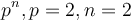# Subgroup structure of Klein four-group

Jump to: navigation, search

## Contents

This article gives specific information, namely, subgroup structure, about a particular group, namely: Klein four-group.
View subgroup structure of particular groups | View other specific information about Klein four-groupWe use here a Klein four-group with identity element$e$ and three non-identity elements$a,b,c$ all of order two.

We can realize this Klein four-group as$\mathbb{Z}/2\mathbb{Z} \times \mathbb{Z}/2\mathbb{Z}$, in which case we can set$a = (1,0), b = (0,1), c = (1,1), e = (0,0)$. For more, see element structure of Klein four-group.

## Tables for quick information

FACTS TO CHECK AGAINST FOR SUBGROUP STRUCTURE: (group of prime power order)
Lagrange's theorem (order of subgroup times index of subgroup equals order of whole group, so all subgroups have prime power orders)|order of quotient group divides order of group (and equals index of corresponding normal subgroup, so all quotients have prime power orders)
prime power order implies not centerless | prime power order implies nilpotent | prime power order implies center is normality-large
size of conjugacy class of subgroups divides index of center
congruence condition on number of subgroups of given prime power order: The total number of subgroups of any fixed prime power order is congruent to 1 mod the prime.

### Quick summary

Item Value
Number of subgroups 5
As elementary abelian group of prime-square order for prime$p = 2$:$p + 3 = 2 + 3 = 5$
Number of conjugacy classes of subgroups 5 (same as number of subgroups, because the group is an abelian group
Number of automorphism classes of subgroups 3
As elementary abelian group of order$p^n, p = 2, n = 2$:$n + 1 = 2 + 1 = 3$
Isomorphism classes of subgroups trivia group (1 time), cyclic group:Z2 (3 times, all in the same automorphism class), Klein four-group (1 time).

### Table classifying subgroups up to automorphism

Note that because abelian implies every subgroup is normal, all the subgroups are normal subgroups.

Automorphism class of subgroups List of subgroups Isomorphism class Order of subgroups Index of subgroups Number of conjugacy classes(=1 iff automorph-conjugate subgroup) Size of each conjugacy class(=1 iff normal subgroup) Total number of subgroups(=1 iff characteristic subgroup) Isomorphism class of quotient (if exists) Subnormal depth Nilpotency class
trivial subgroup$\{ e \}$ trivial group 1 4 1 1 1 Klein four-group 1 0
Z2 in V4$\{ e, a\}, \{ e,b \}, \{ e,c \}$ cyclic group:Z2 2 2 3 1 3 cyclic group:Z2 1 1
whole group$\{ e,a,b,c\}$ Klein four-group 4 1 1 1 1 trivial group 0 1
Total (3 rows) -- -- -- -- 5 -- 5 -- -- --